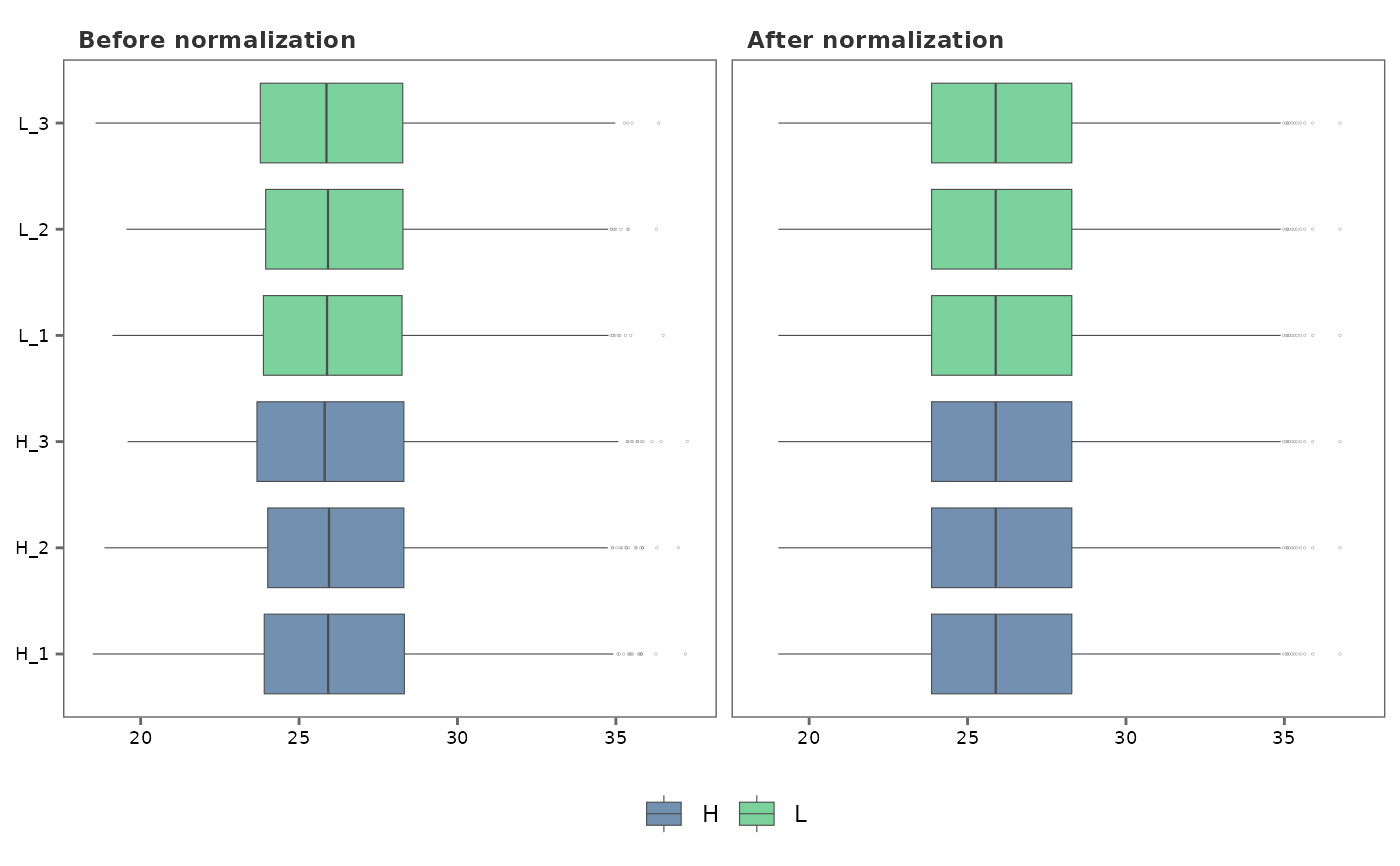This function visualizes the impact of normalization on the data

## Usage

norm_plot(
original,
normalized,
type = "box",
text_size = 10,
palette = "viridis",
save = FALSE,
file_path = NULL,
file_name = "Norm_plot",
file_type = "pdf",
dpi = 80,
plot_width = 10,
plot_height = 7
)

## Arguments

original

A raw_df object (output of create_df) containing missing values, or an imp_df object after imputing the missing values with impute_na.

normalized

A norm_df object after normalizing the data frame provided as original using normalize_data.

type

Type of plot to generate. Choices are "box" or "density." Default is "box."

text_size

Text size for plot labels, axis labels etc. Default is 10.

palette

Viridis color palette option for plots. Default is "viridis". See viridis for available options.

save

Logical. If TRUE saves a copy of the plot in the directory provided in file_path.

file_path

A string containing the directory path to save the file.

file_name

File name to save the plot. Default is "Norm_plot."

file_type

File type to save the plot. Default is "pdf".

dpi

Plot resolution. Default is 80.

plot_width

Width of the plot. Default is 10.

plot_height

Height of the plot. Default is 7.

## Value

A ggplot2 plot object.

## Details

Given two data frames, one with data prior to normalization (original), and the other, after normalization (normalized), norm_plot generates side-by-side plots to visualize the effect of normalization on the protein intensity data.

• create_df

• impute_na

## Author

Chathurani Ranathunge

## Examples

# \donttest{
## Generate a raw_df object with default settings. No technical replicates.
raw_df <- create_df(
prot_groups = "https://raw.githubusercontent.com/caranathunge/promor_example_data/main/pg1.txt",
exp_design = "https://raw.githubusercontent.com/caranathunge/promor_example_data/main/ed1.txt"
)
#> 0 empty row(s) removed.
#> 0 empty column(s) removed.
#> 80 protein(s) (rows) only identified by site removed.
#> 65 reverse protein(s) (rows) removed.
#> 42 protein potential contaminant(s) (rows) removed.
#> 1923 protein(s) identified by 2 or fewer unique peptides removed.
#> Zeros have been replaced with NAs.
#> Data have been log-transformed.

## Impute missing values in the data frame using the default minProb
## method.
imp_df <- impute_na(raw_df)

## Normalize the imp_df object using the default quantile method
norm_df <- normalize_data(imp_df)

## Visualize normalization using box plots
norm_plot(original = imp_df, normalized = norm_df)
#> Warning: argument is not numeric or logical: returning NA
#> Warning: argument is not numeric or logical: returning NA
#> Warning: argument is not numeric or logical: returning NA
#> Warning: argument is not numeric or logical: returning NA
#> Warning: argument is not numeric or logical: returning NA
#> Warning: argument is not numeric or logical: returning NA
#> Warning: argument is not numeric or logical: returning NA
#> Warning: argument is not numeric or logical: returning NA
#> Warning: argument is not numeric or logical: returning NA
#> Warning: argument is not numeric or logical: returning NA
#> Warning: argument is not numeric or logical: returning NA
#> Warning: argument is not numeric or logical: returning NA## Visualize normalization using density plots
norm_plot(imp_df, norm_df, type = "density")# }